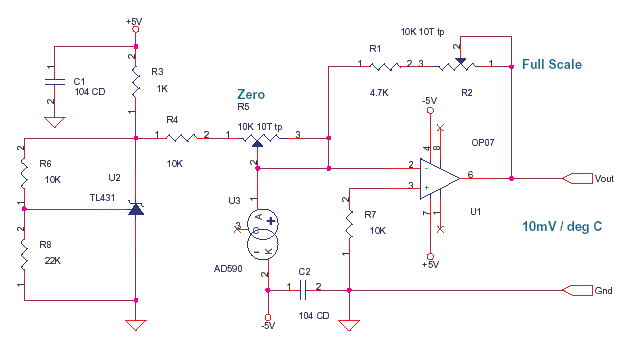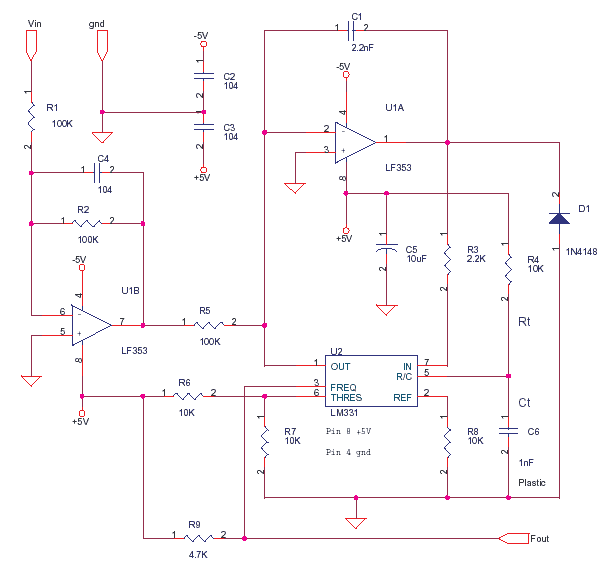## Schematics of delabs

One of the oldest Schematic Archives on the Web. It was in the form of pdf files in the late 90s. Instrumentation and Industrial Designs by delabs and Generic Application Designs.

## Tuesday, September 22, 2015

Learn how to use the AD590 to measure environment temperatures for display, logging or cold junction compensation.﻿

The voltage at the point 1 of R4 will be :Vo=( 1+ ( 10K/22K)) * Vref = 3.63V as nominal Vref is
2.5V.AD590 is a current source which gives 1 uA / kelvin, It is independent of the voltage across the device. you can treat it like a current source or sink or impedance. total voltage across AD590 is 5V as opamp pin 2 is at virtual ground.

Analog Circuits - OpAmp, Signal Condition, Mixed Signal.This is the way you try to understand the design.

The AD590, here is a constant current sink as cathode goes to -5. The current it sucks away or drains from node pin 2 of OP07 is 1uA/ kelvin. at 0 deg C the current drained is 273 uA at 26 deg C it is 300uA.

You know according to theory that the amount of current entering the node, is equal to the amount of current leaving the node. do not look at voltages now, look at the currents. the AD590 drinks 273uA from Node pin 2 of OP07 at 0 deg C. Now no current can come from opamp OP07 pin 2 as resistance is in giga ohms and leakage in pico amps. now the pot R5 and resistor R4 are just in series and connected to 3.63 V as established earlier. The TL431 is a shunt regulator with reference and has a low impedence. Now the R5 + R4 combination should not load the TL431, that is not the case as 3.6 / 10K = 360uA .

By varying R5 pot you can pump 3.6 / 10K = 360uA down to 130uA when R5 is max into node pin 2 of OP07. This pot will be calibrated with AD590 in ICE to give a 0 mV output of the Op07. When calibrated R5+R4 pump 273 uA into node pin 2 of op07. this is sucked away by the AD590 which is draining 273uA at 0 deg C. This leaves the pin 2 at zero potential as currents leaving = currents entering.

Now to understand the opamp functioning.

The pin 2 of opamp is a 0 potential as calculated above and pin 3 also is at zero pulled down by R7. Now as both inputs are at same potential the output of opamp also is zero. The feedback resistors R1 and R2 will carry no current as both their ends are at 0. the Vout is now 0 mV and AD590 is on a block of ICE and opamp is stable.

If pin 2 (-) becomes more dominant or positive than pin 3 (+) the output swings negative. If pin 3 (+) becomes more dominant or positive than pin 2 (-) the output swings positive. The opamp on feedback tries to maintain both the inputs at the same potential. This thumb rule can be used to make opamp oscillate, amplify or compute.

Now what happens when the AD590 is removed from the block of ICE. It comes to room temperature say 26 deg C which means 300uA. Now the AD590 demands to draw 300uA from node pin 2 of OP07. The R4 + R5 from 3.6 V can give 273uA as it is fixed, not a uA more. The rest which is 300 - 273 = 27uA leads to a drop in potential at pin 2 and it turns negative. as demand is greater than supply. which makes pin 3 which is at zero more positive than pin 2. ( theory : 0 is positive compared to -1) as pin 3 is more dominant opamp swings positive as per thumb rule. and a current starts flowing thru R1 + R2 till the current reaches 27uA. at this point the extra current 27uA drawn by AD590 is supplied by opamp thru R1+R2. The Pin 2 now comes to 0 as currents leaving = currents entering.

Test & Measurement, Instrumentation

At this point the voltage at opamp output is given by ( R1 + R2 ) * 27uA = 270mV (assume R1+R2 is 10K after calibration) now opamp gives 10mV per deg C.as opamp now is a closed loop control the rise and fall in temperature, results in AD590 current variation which produces a proportional OP07 output.

Now the explanation above is in steps but all that happens in real time in an instant.

## Monday, September 21, 2015

### FET Current Source and MOSFET

This is a constant current source using a FET. This is the most simple replacement to series resistor to limit current. The N-Channel FET BF256C can give 15mA current.Simple Methods

Before you get to use chips, experiment with some methods, which will help you learn about the LEDs better. The first is just One Resistor in series. This is to Limit the max current in a Series LED Chain. If you have a Regulated Supply with a Fixed Voltage, then you can use this method.

Let us take a 12V SMPS, Each HB White LED has a drop of around 3.2 (please see datasheet). If you put 3 LEDs in series it is a drop of 3 X 3.2 = 9.6 V.

12V - 9.6V = 2.4 V. This is the drop across the Resistor, let us keep the current at 20mA for a Long life for LED. Some LEDs will get damaged at 30mA some take more that that. We now have LED Modules which can take even 1 or 2 A.V/I = R as per OHM. 2.4V/20mA = 120 E or Ohms.

How Hot? W = VI Power in Watts. 20mA x 2.4V = 48mW. This is where you lose the Money. Keep it low, else the Green Goblin will frown. Unless you want LED Lighting to double up as a Room Heater, Nice idea if you are in the Artic.

Now you have a chain of 3 LED with one R, make many such chains and put it in parallel to around 70% of SMPS capacity. If you have 20 Strips of 3 LEDs each, 20 X 20mA = 400mA. You will need a 12V 600mA SMPS .

MOSFET Drive for LED Constant Current

Let us assume, you have a supply that is varying and not stable. Then use a SMPS and Resistor as shown above. Closely matching the LED Chain to the SMPS voltage to keep the heat loss minimum. At Low voltages the above idea may not work. So you can try a MOSFET circuit shown.

You can use Transistors too but The Heat is more, as the Drop is more. When you use batteries, you cannot afford to lose even 0.5V. So the MOSFET is the answer.

This circuit is a nice design idea about LED drive with low voltage and watts burden. In combination with a Joule Thief - and PWM you can make many White LED utilities like Lanterns and Flashlights. PWM is to modulate brightness and also Extend LED Life.

The Essence is The LED has to have a long life, constant current is the answer. The Efficiency has to be High, Switching MOSFET is the answer. For just a LED or Two, you do not need to bother about Efficiency, but Constant Current, No Compromise. Why? Because it is in the Absolute Maximum Rating."You do not cross the road, when the light is RED. You do not Drive Faster, than the Speed Limit. You do not Eat, more than you can Digest.You do not Stress the Absolute Max in the Ratings."

The Elektrik Jedi

## Sunday, September 06, 2015

### VCO with LM331

This Circuit is a simple Analog to Digital Interface with a capability of 10 to 12 bits resolution. 10 bits means 1024 counts or parts of a full scale FS which is close to 3-1/2 1999 counts.In this 1 V can be read as 1.000 V that means even 1mV can be resolved for FS of 1V. The Caps C6 and others must be plastic multilayer low-leakage types for accuracy of reading. Use all 1% MFR 100ppm or better resistors, Design gain of U1B for the Full Scale you want.

Mixed Circuits Analog with Digital

The Output Fout is a Frequency which is directly proportional to the measured voltage Vin. The pulses can be isolated using opto-couplers to avoid ground loops or electric hazard. An additional protection and scaling circuit at the input may be required for some sensors.